Prophet简介

• 趋势：宏观、长期、持续性的作用力
• 周期：比如商品价格在较短时间内，围绕某个均值上下波动；
• 季节：变化规律相对固定，并呈现某种周期特征。“季节”不一定按年计。每周、每天的不同时段的规律，也可称作季节性。
• 随机：随机的不确定性，也是人们常说的随机过程 (Stochastic Process）。

• 观察值是按每小时或每天或每周或每月给出的一段历史数据
• 多尺度的周期性：一周七天，一个月30天，12个月等等
• 提前已知的一些重要假期：各种法定节日或传统节日
• 数据中存在缺失值或异常值
• 历史趋势的变化
• 趋势是非线性变化，达到自然极限或趋于饱和

Prophet通过将全自动预测与在线学习相结合从而保证了该工具能够解决大多数商业业务问题，Prophet工作流程如下图所示：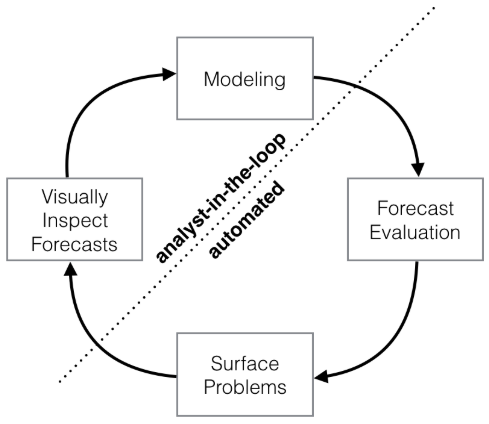• Modeling：建立时间序列模型。分析师根据预测问题的背景选择一个合适的模型。
• Forecast Evaluation：模型评估。根据模型对历史数据进行仿真，在模型的参数不确定的情况下，我们可以进行多种尝试，并根据对应的仿真效果评估哪种模型更适合。
• Surface Problems：呈现问题。如果尝试了多种参数后，模型的整体表现依然不理想，这个时候可以将误差较大的潜在原因呈现给分析师。
• Visually Inspect Forecasts：以可视化的方式反馈整个预测结果。当问题反馈给分析师后，分析师考虑是否进一步调整和构建模型。

• Prophet能够让你更方便直接地创建一个预测任务，而其他的一些工具包（ARMA，指数平滑）等，这些工具每个有自己的优缺点及参数，即使是优秀的数据分析师想要从众多的模型中选择合适的模型及相应的参数也是够让他头皮发麻的。
• Prophet是为非专家们“量身定制”的。为什么这么说呢？你利用Prophet可以直接通过修改季节参数来拟合季节性，修改趋势参数来拟合趋势信息，指定假期来拟合假期信息等等。

Prophet实例解析

Prophet遵循sklearn模型API。 我们可以创建一个prophet类的实例，然后调用它的fit和predict方法。 Prophet的输入必须包含两列数据：ds和y，其中ds是时间戳列，必须是时间信息；y列必须是数值，代表我们需要预测的信息。我们使用官方示例中的数据进行讲解。

import pandas as pd
from fbprophet import Prophet

m = Prophet()
m.fit(df)
future = m.make_future_dataframe(periods=365)
forecast = m.predict(future)
forecast[['ds', 'yhat', 'yhat_lower', 'yhat_upper']].tail()
fig1 = m.plot(forecast)
fig2 = m.plot_components(forecast)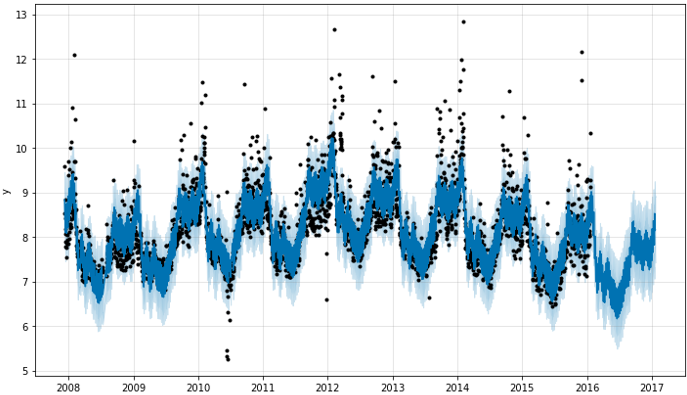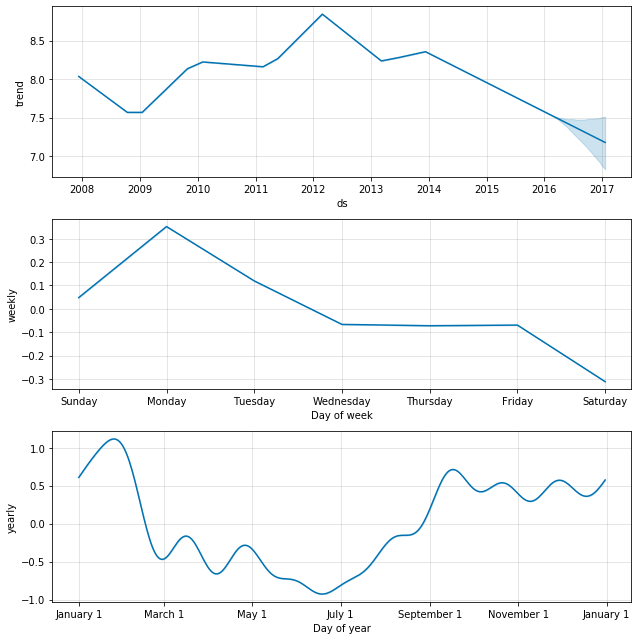Prophet的官网上本身没有对API的进一步说明。我们只能从代码入手：

class Prophet(object):
"""Prophet forecaster.

Parameters
----------
growth: String 'linear' or 'logistic' to specify a linear or logistic
trend.
changepoints: List of dates at which to include potential changepoints. If
not specified, potential changepoints are selected automatically.
n_changepoints: Number of potential changepoints to include. Not used
if input changepoints is supplied. If changepoints is not supplied,
then n_changepoints potential changepoints are selected uniformly from
the first changepoint_range proportion of the history.
changepoint_range: Proportion of history in which trend changepoints will
be estimated. Defaults to 0.8 for the first 80%. Not used if
changepoints is specified.
Not used if input changepoints is supplied.
yearly_seasonality: Fit yearly seasonality.
Can be 'auto', True, False, or a number of Fourier terms to generate.
weekly_seasonality: Fit weekly seasonality.
Can be 'auto', True, False, or a number of Fourier terms to generate.
daily_seasonality: Fit daily seasonality.
Can be 'auto', True, False, or a number of Fourier terms to generate.
holidays: pd.DataFrame with columns holiday (string) and ds (date type)
and optionally columns lower_window and upper_window which specify a
range of days around the date to be included as holidays.
lower_window=-2 will include 2 days prior to the date as holidays. Also
optionally can have a column prior_scale specifying the prior scale for
that holiday.
seasonality_prior_scale: Parameter modulating the strength of the
seasonality model. Larger values allow the model to fit larger seasonal
fluctuations, smaller values dampen the seasonality. Can be specified
holidays_prior_scale: Parameter modulating the strength of the holiday
components model, unless overridden in the holidays input.
changepoint_prior_scale: Parameter modulating the flexibility of the
automatic changepoint selection. Large values will allow many
changepoints, small values will allow few changepoints.
mcmc_samples: Integer, if greater than 0, will do full Bayesian inference
with the specified number of MCMC samples. If 0, will do MAP
estimation.
interval_width: Float, width of the uncertainty intervals provided
for the forecast. If mcmc_samples=0, this will be only the uncertainty
in the trend using the MAP estimate of the extrapolated generative
model. If mcmc.samples>0, this will be integrated over all model
parameters, which will include uncertainty in seasonality.
uncertainty_samples: Number of simulated draws used to estimate
uncertainty intervals.
"""

def __init__(
self,
growth='linear',
changepoints=None,
n_changepoints=25,
changepoint_range=0.8,
yearly_seasonality='auto',
weekly_seasonality='auto',
daily_seasonality='auto',
holidays=None,
seasonality_prior_scale=10.0,
holidays_prior_scale=10.0,
changepoint_prior_scale=0.05,
mcmc_samples=0,
interval_width=0.80,
uncertainty_samples=1000,
):

Prophet()参数说明：

• growth: 字符串‘linear’或‘logistic’，表示线性或者逻辑增长趋势。
• changepoints: 日期型向量，指定潜在拐点，如果不指定，将会自动选择潜在拐点。例如：changepoints=[‘2014-01-01’]指定2014-01-01这一天是潜在的changepoints。
• n_changepoints: 表示changepoints的数量大小，如果changepoints指定，该传入参数将不会被使用。如果 changepoints不指定，将会从输入的历史数据前80%中选取25个（个数由n_changepoints传入参数决定）潜在改变点。
• changepoint_range: 估计趋势变化点的历史比例。如果指定了 changepoints，则不使用。
• yearly_seasonality: 指定是否分析数据的年季节性，如果为True，默认取傅里叶项为10，最后会输出，yearly_trend,yearly_upper,yearly_lower等数据。
• weekly_seasonality: 指定是否分析数据的周季节性，如果为True，默认取傅里叶项为10，最后会输出，weekly_trend,weekly_upper,weekly_lower等数据。
• daily_seasonality: 指定是否分析数据的天季节性，如果为True，默认取傅里叶项为10，最后会输出，daily_trend, daily_upper, daily_lower等数据。
• holidays: 传入dataframe 格式的数据。这个数据包含有holiday列 (string)和ds(date类型）和可选列lower_window和upper_window来指定该日期的lower_window或者upper_window范围内都被列为假期。lower_window=-2将包括前2天的日期作为假期。（默认None）
• seasonality_mode: ‘additive’ (default) or ‘multiplicative’。季节模型。
• seasonality_prior_scale: 调节季节性组件的强度。值越大，模型将适应更强的季节性波动，值越小，越抑制季节性波动。
• holidays_prior_scale: 调节节假日模型组件的强度。值越大，该节假日对模型的影响越大，值越小，节假日的影响越小。
• changepoint_prior_scale: 增长趋势模型的灵活度。调节“changepoint”选择的灵活度，值越大选择的“changepoint”越多，使模型对历史数据的拟合程度变强，然而也增加了过拟合的风险。
• mcmc_samples: mcmc采样，用于获得预测未来的不确定性。若大于0，将做mcmc样本的全贝叶斯推理，如果为0，将做最大后验估计。
• interval_width: 衡量未来时间内趋势改变的程度。表示预测未来时使用的趋势间隔出现的频率和幅度与历史数据的相似度，值越大越相似。当mcmc_samples = 0时，该参数仅用于增长趋势模型的改变程度，当mcmc_samples > 0时，该参数也包括了季节性趋势改变的程度。
• uncertainty_samples: 用于估计不确定性区间的模拟抽取数
def make_future_dataframe(self, periods, freq='D', include_history=True):
"""Simulate the trend using the extrapolated generative model.

Parameters
----------
periods: Int number of periods to forecast forward.
freq: Any valid frequency for pd.date_range, such as 'D' or 'M'.
include_history: Boolean to include the historical dates in the data
frame for predictions.

Returns
-------
pd.Dataframe that extends forward from the end of self.history for the
requested number of periods.
"""

make_future_dataframe()参数说明：

• periods: 向前预测步数
• freq: 预测单位小时为’H’，天为’D’，月为’M’
• include_history: 是否包含历史数据的预测

Prophet的算法实现

$$y(t)=g(t)+s(t)+h(t)+\epsilon_t$$

• g(t)：表示趋势项，它表示时间序列在非周期上面的变化趋势。
• s(t)：表示周期项，或者称为季节项，一般来说是以周或者年为单位。
• h(t)：表示节假日项，表示在当天是否存在节假日。
• $\epsilon_t$：表示误差项或者称为剩余项。

Prophet 算法就是通过拟合这几项，然后最后把它们累加起来就得到了时间序列的预测值。

趋势项$g(t)$

$$f(x) = \frac{C}{1+e^{-k(x-m)}}$$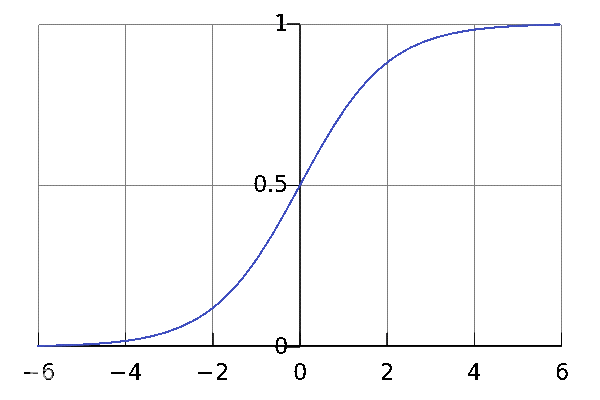$$\frac{y^{\prime}}{y}+\frac{y^{\prime}}{1-y}=1 \Rightarrow \ln \frac{y}{1-y}=1 \Rightarrow y=1 /\left(1+K e^{-x}\right)$$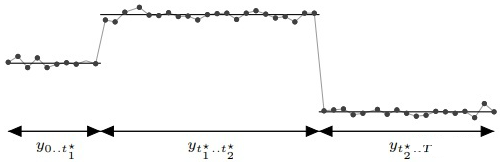$$a_{j}(t)=\left\{\begin{array}{ll}{1,} & {\text { if } t \geq s_{j}} \\ {0,} & {\text { otherwise }}\end{array}\right.$$

$$\gamma_{j}=\left(s_{j}-m-\sum_{\ell<j} \gamma_{\ell}\right) \cdot\left(1-\frac{k+\sum_{\ell<j} \delta_{\ell}}{k+\sum_{\ell \leq j} \delta_{\ell}}\right)$$

$$g(t) = \frac{C(t)}{1+\exp (-(k+a(t)^t \delta ))\cdot (t-(m+a(t)^T\gamma ))}$$

Prophet可以主动识别两个参数：’cap’和’floor’，其中’cap’为必传参数，没有默认值。’floor’非必传，默认值为0。示例代码：

import pandas as pd
from fbprophet import Prophet

df['cap'] = 10.8  # 上限
df['floor'] = 6  # 下限
m = Prophet(growth='logistic')
m.fit(df)
future = m.make_future_dataframe(periods=365)
future['cap'] = 10.8  # 必须一起添加
future['floor'] = 6
forecast = m.predict(future)
forecast[['ds', 'yhat', 'yhat_lower', 'yhat_upper']].tail()
fig1 = m.plot(forecast)$$g(t)=(k+a(t)\delta )\cdot t + (m+a(t)^T\gamma )$$

growth=’linear’和growth=’logistic’该如何选择？

$$f(x | \mu, b)=\frac{\exp (-\frac{|x-\mu|}{b})}{2b}$$

• changepoint_range，指的是百分比，需要在前changepoint_range那么长的时间序列中设置变点，在默认的函数中是 changepoint_range = 0.8。
• n_changepoint，表示变点的个数，在默认的函数中是 n_changepoint = 25。
• changepoint_prior_scale，表示变点增长率的分布情况，在论文中，$\delta_{j} \sim \text {Laplace}(0, \tau)$，这里的$\tau$就是 change_point_scale。

changepoint_ts_new = 1 + np.random.rand(n_changes) * (T - 1)
changepoint_ts = np.concatenate((self.changepoints_t, changepoint_ts_new))

$$\delta_{j}= \{\begin{array}{l}{0, \text { with probability }(T-S) / T} \\ {\sim \text {Laplace}(0, \lambda), \text { with probability } S / T}\end{array}$$

周期（季节）项$s(t)$

$$s(t)=\sum_{n=1}^{N}(a_{n} \cos (\frac{2 \pi n t}{P})+b_{n} \sin (\frac{2 \pi n t}{P}))$$

模型拟合（Model Fitting）

$$y(t)=g(t)+s(t)+h(t)+\epsilon_t$$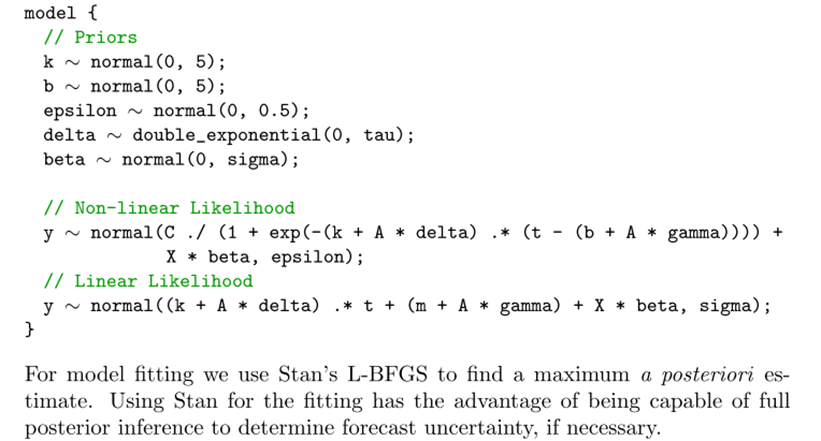• 分段线性或逻辑增长曲线趋势。 Prophet通过从数据中选择改变点，自动检测趋势的变化。
• 用傅里叶级数模拟每年的季节性分量。
• 用虚拟变量来模拟每周的周期性分量。
• 用户提供的重要节日列表

Prophet其中最重要的思想就是曲线拟合，这与传统的时序预测算法有很大的不同。

Prophet的置信区间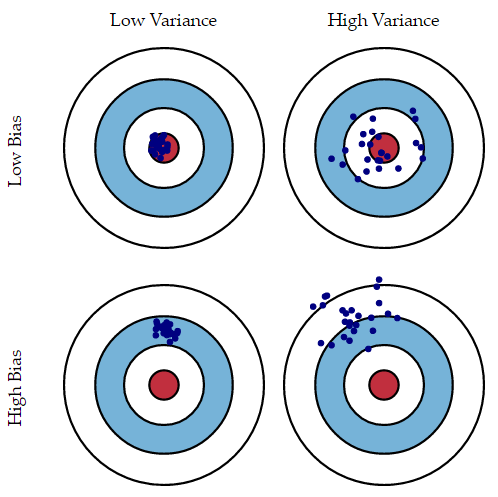my_model = Prophet(interval_width=0.95) #设置置信空间为95%(如果不设置的话默认80%)
my_model.fit(data)

my_model = Prophet(interval_width=0.95, mcmc_samples=500)
my_model.fit(data)

Prophet的安装

fbprophet为Prophet在Python环境下的包，想要使用fbprohhet并没有想象中的那么简单，特别是在Windows系统上可能发生错误。主要原因是fbprophet基于pystan，pystan基于cython。问题会卡在pystan的安装上。

pip install cython
pip install pystan
pip install fbprophet

PyStan is partially supported under Windows with the following caveats:

Python 2.7: Doesn’t support parallel sampling. When drawing samples n_jobs=1 must be used)

Python 3.5 or higher: Parallel sampling is supported

MSVC compiler is not supported.

PyStan requires a working C++ compiler. Configuring such a compiler is typically the most challenging step in getting PyStan running.

PyStan is tested against the MingW-w64 compiler which works on both Python versions (2.7, 3.x) and supports x86 and x64.

Due to problems with MSVC template deduction, functions with Eigen library are failing. Until this and other bugs are fixed no support is provided for Windows + MSVC. Currently, no fix is known for this problem, other than to change the compiler to GCC or clang-cl.

• 下载MingW-w64，并进行安装，下载地址：https://osdn.net/projects/mingw/releases/
• 将mingw的路径添加到环境变量的PATH中，示例路径：C:\mingw-w64\x86_64-7.1.0-posix-seh-rt_v5-rev0\mingw64\bin
• 验证编译环境是否OK，验证方式为在cmd中执行如下命名 gcc –dumpversion、ld –v、dllwrap –version
• 修改Python内部编译设置，方法为在Python安装目录下（示例：C:\Python36\Lib\distutils），新建distutils.cfg文件，文件内容为
[build]
compiler = mingw32

conda update conda
conda install libpython m2w64-toolchain -c msys2

Linux下报ModuleNotFoundError: No module named ‘pystan’ 的解决方案：

pip uninstall pystan
pip install pystan~=2.14
pip install fbprophet

update：无需安装编译器

pip install pystan==2.17.1.0
pip install fbprophet==0.6

Prophet调参经验

• 首先我们去除数据中的异常点（outlier），直接赋值为none就可以，因为Prophet的设计中可以通过插值处理缺失值，但是对异常值比较敏感。
• 选择趋势模型，默认使用分段线性的趋势，但是如果认为模型的趋势是按照log函数方式增长的，可设置growth=’logistic’从而使用分段log的增长方式
• 设置趋势转折点（changepoint），如果我们知道时间序列的趋势会在某些位置发现转变，可以进行人工设置，比如某一天有新产品上线会影响我们的走势，我们可以将这个时刻设置为转折点。
• 设置周期性，模型默认是带有年和星期以及天的周期性，其他月、小时的周期性需要自己根据数据的特征进行设置，或者设置将年和星期等周期关闭。
• 设置节假日特征，如果我们的数据存在节假日的突增或者突降，我们可以设置holiday参数来进行调节，可以设置不同的holiday，例如五一一种，国庆一种，影响大小不一样，时间段也不一样。
• 此时可以简单的进行作图观察，然后可以根据经验继续调节上述模型参数，同时根据模型是否过拟合以及对什么成分过拟合，我们可以对应调节seasonality_prior_scale、holidays_prior_scale、changepoint_prior_scale参数。
• 如果预测结果的误差很大，考虑选取的模型是否准确，尝试调整增长率模型（growth）的参数，在必要的情况下也需要调整季节性（seasonality）参数。
• 如果在尝试的大多数方法中，某些日期的预测依然存在很大的误差，这就说明历史数据中存在异常值。最好的办法就是找到这些异常值并剔除掉。使用者无需像其他方法那样对剔除的数据进行插值拟合，可以仅保留异常值对应的时间， 并将异常值修改为空值（NA），模型在预测时依然可以给出这个时间点对应的预测结果。
• 如果对历史数据进行仿真预测时发现，从一个截点到下一个截点误差急剧的增加，这说明在两个截点期间数据的产生过程发生了较大的变化，此时两个截点之间应该增加一个”changepoint”，来对这期间的不同阶段分别建模。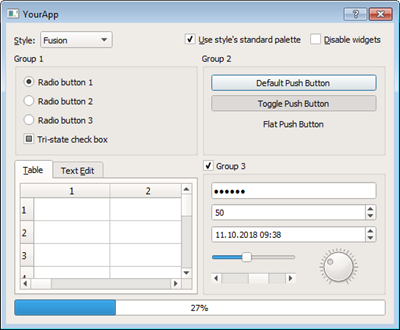Python图形界面框架PyQt5AppStore 搜索竞价广告ASA